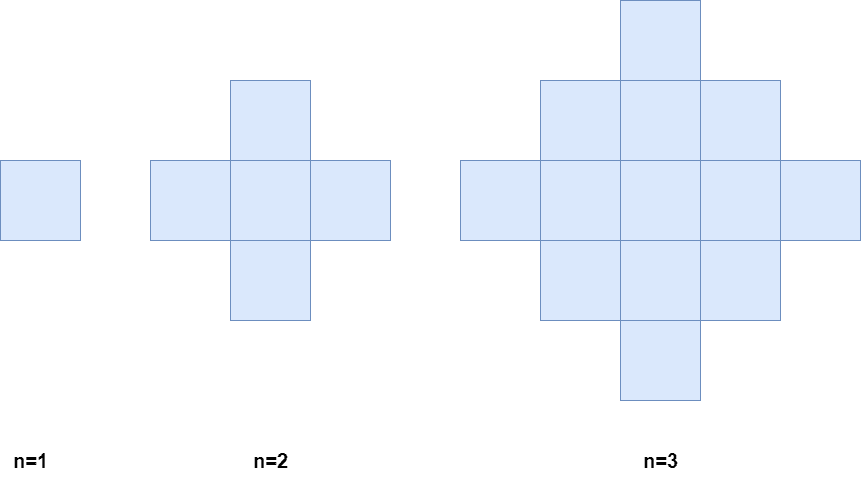##### Welcome to Subscribe On Youtube

Formatted question description: https://leetcode.ca/all/2579.html

# 2579. Count Total Number of Colored Cells

## Description

There exists an infinitely large two-dimensional grid of uncolored unit cells. You are given a positive integer n, indicating that you must do the following routine for n minutes:

• At the first minute, color any arbitrary unit cell blue.
• Every minute thereafter, color blue every uncolored cell that touches a blue cell.

Below is a pictorial representation of the state of the grid after minutes 1, 2, and 3.Return the number of colored cells at the end of n minutes.

Example 1:

Input: n = 1
Output: 1
Explanation: After 1 minute, there is only 1 blue cell, so we return 1.


Example 2:

Input: n = 2
Output: 5
Explanation: After 2 minutes, there are 4 colored cells on the boundary and 1 in the center, so we return 5.


Constraints:

• 1 <= n <= 105

## Solutions

• class Solution {
public long coloredCells(int n) {
return 2L * n * (n - 1) + 1;
}
}

• class Solution {
public:
long long coloredCells(int n) {
return 2LL * n * (n - 1) + 1;
}
};

• class Solution:
def coloredCells(self, n: int) -> int:
return 2 * n * (n - 1) + 1


• func coloredCells(n int) int64 {
return int64(2*n*(n-1) + 1)
}

• function coloredCells(n: number): number {
return 2 * n * (n - 1) + 1;
}


• impl Solution {
pub fn colored_cells(n: i32) -> i64 {
2 * (n as i64) * (n as i64 - 1) + 1
}
}